Next: Conclusions Up: Grammatical Evolution : Solving Previous: The Problem Space

# Results

GE successfully found the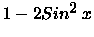identity for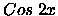on the majority of runs. An example of how one solution was arrived at will now be given. Early on in the run very simple expressions existed, such as 1.0 which had a fitness 0.08 (The best fitness an individual may have is 1.0),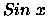whose fitness was 0.07,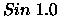whose fitness was 0.10, and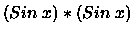whose fitness was 0.06, while not particularly fit these individuals are the essential building blocks for the ultimate solution.

After the first 20 generations the best individuals were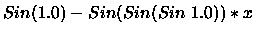which had a fitness 0.08,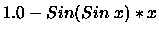which had a fitness 0.13,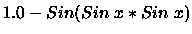which had a fitness 0.15.

Shortly after this, two individuals appeared that gradually spread amongst the population. These were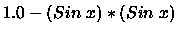,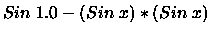,

whose fitness were 0.17 and 0.20 respectively. Again, while not particularly fit themselves, they are important steps on the path to the solution. All that was needed now was another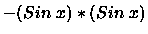to be added onto the first of these expressions. Generation 39 saw this come to pass with a slight variation, the individual being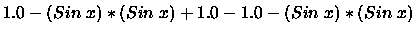,

which had the fitness of 1.00.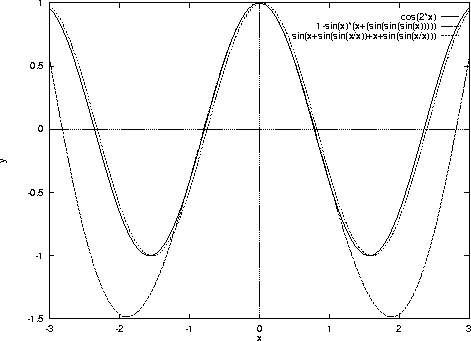Subsequent runs produced other notable individuals. One individual seen in Figure 3,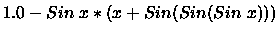,

approximated the functionvery closely in the range -1 to +1 but outside this range it deviated from. Others resembled the target functions overall appearance almost exactly, but were phase shifted away by a very small number of radians, one of these individuals seen in Figure 3 was

Sin(x+Sin(Sin(x/x))+x+Sin(Sin(x/x))).

Another interesting individual

Sin(x+x+1.0/Sin(Sin(Sin(Sin(1.0))))),

seen in Figure 4, was almost identical to the target function. Interestingly, in this individual we can see the successive application of the Sin function to the constant 1.0. Koza also observed a similar phenomenon with Genetic Programming [Koza 92]. Effectively GE is evolving a numerical constant. Successive application of the Sin function to 1.0 results in a constantly decreasing value. After four applications of Sin to 1.0 the result is 0.628, and then the subsequent reciprocal produces 1.59. This is close to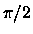which is approximately 1.57. Simplified the expression can now be shown as follows: Sin(2x+1.59) , which is very close to another well known indentity of, that being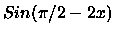.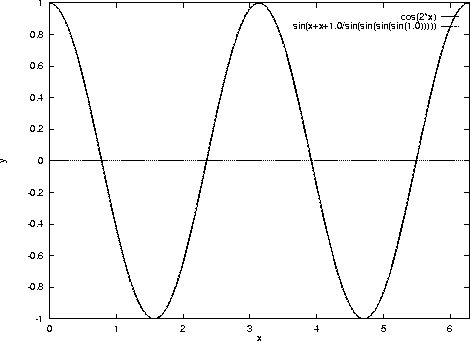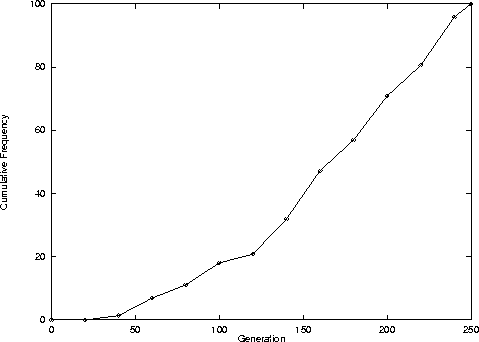Figure 5 shows a cumulative frequency measure of when the solution was arrived at. When compared with Genetic Programming, GE is somewhat slower at generating the solution for this particular problem. We are not unduly concerned at GP being faster, as we don't expect GE to be viewed as a replacement to GP. Rather, that it complements GP, and is intended to be used for the generation of functions of arbitray complexity. We are more concerned with proving that this system can generate functions of this type. It is envisaged that when it comes to problems requiring multi-line function solutions, GE will come into it's own.Next: Conclusions Up: Grammatical Evolution : Solving Previous: The Problem Space
root
1998-10-02